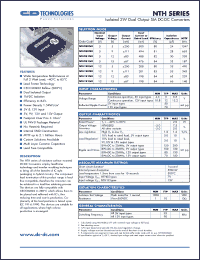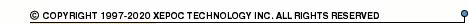More than12 563 976 queries processedNTH series datasheets. Manufacturer: CANDD.

 NTH0505M Isolated 2W dual output SM DC-DC converter. Nom.input voltage 5V, output voltage 5V, output current +-200mA. in 26-pin SMD package. Operational temperature range from -40°C to 85°C. Datasheet*) NTH0505M-R Isolated 2W dual output SM DC-DC converter. Nom.input voltage 5V, output voltage 5V, output current +-200mA. in 26-pin SMD package. Operational temperature range from -40°C to 85°C. Datasheet*) NTH0509M Isolated 2W dual output SM DC-DC converter. Nom.input voltage 5V, output voltage 9V, output current +-111mA. in 26-pin SMD package. Operational temperature range from -40°C to 85°C. Datasheet*) NTH0509M-R Isolated 2W dual output SM DC-DC converter. Nom.input voltage 5V, output voltage 9V, output current +-111mA. in 26-pin SMD package. Operational temperature range from -40°C to 85°C. Datasheet*) NTH0512M Isolated 2W dual output SM DC-DC converter. Nom.input voltage 5V, output voltage 12V, output current +-83mA. in 26-pin SMD package. Operational temperature range from -40°C to 85°C. Datasheet*) NTH0512M-R Isolated 2W dual output SM DC-DC converter. Nom.input voltage 5V, output voltage 12V, output current +-83mA. in 26-pin SMD package. Operational temperature range from -40°C to 85°C. Datasheet*) NTH0515M Isolated 2W dual output SM DC-DC converter. Nom.input voltage 5V, output voltage 15V, output current +-67mA. in 26-pin SMD package. Operational temperature range from -40°C to 85°C. Datasheet*) NTH0515M-R Isolated 2W dual output SM DC-DC converter. Nom.input voltage 5V, output voltage 15V, output current +-67mA. in 26-pin SMD package. Operational temperature range from -40°C to 85°C. Datasheet*) NTH1205M Isolated 2W dual output SM DC-DC converter. Nom.input voltage 12V, output voltage 5V, output current +-200mA. in 26-pin SMD package. Operational temperature range from -40°C to 85°C. Datasheet*) NTH1205M-R Isolated 2W dual output SM DC-DC converter. Nom.input voltage 12V, output voltage 5V, output current +-200mA. in 26-pin SMD package. Operational temperature range from -40°C to 85°C. Datasheet*) NTH1209M Isolated 2W dual output SM DC-DC converter. Nom.input voltage 12V, output voltage 9V, output current +-111mA. in 26-pin SMD package. Operational temperature range from -40°C to 85°C. Datasheet*) NTH1209M-R Isolated 2W dual output SM DC-DC converter. Nom.input voltage 12V, output voltage 9V, output current +-111mA. in 26-pin SMD package. Operational temperature range from -40°C to 85°C. Datasheet*) NTH1212M Isolated 2W dual output SM DC-DC converter. Nom.input voltage 12V, output voltage 12V, output current +-83mA. in 26-pin SMD package. Operational temperature range from -40°C to 85°C. Datasheet*) NTH1212M-R Isolated 2W dual output SM DC-DC converter. Nom.input voltage 12V, output voltage 12V, output current +-83mA. in 26-pin SMD package. Operational temperature range from -40°C to 85°C. Datasheet*) NTH1215M Isolated 2W dual output SM DC-DC converter. Nom.input voltage 12V, output voltage 15V, output current +-67mA. in 26-pin SMD package. Operational temperature range from -40°C to 85°C. Datasheet*) NTH1215M-R Isolated 2W dual output SM DC-DC converter. Nom.input voltage 12V, output voltage 15V, output current +-67mA. in 26-pin SMD package. Operational temperature range from -40°C to 85°C. Datasheet*) NTH Isolated 2W Dual Output SM DC-DC Converters Datasheet*)